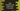# Java program to find pairs with a given sum in an array## Java program to find pairs with a given sum in an array :

### Problem :

One array is given with unsorted numbers. Find the total number of pairs of elements, the sum of which is equal to a given value :

### Solution :

1. We will scan the array two times.

2. First of all, create one empty HashMap. This HashMap is used to store the numbers as keys and count for each number in the array as value for each one.

3. On its first iteration, check if any key exists or not in the hashmap, that is equal to the current iterating element of the array.

4. If it doesn’t exist, add it to the HashMap with its value as 1

5. For the array {1,2,3,4,5,4,3} , the map(key,value) will be like 1 : 1, 2 : 1, 3 : 2 ,4 : 2, 5 : 1

6. On second iteration, check if any key that is equal to the (sum - element) exists in the map or not. If it exists, increment the count variable by one and delete the key element.

7. Initialize one varible as 0 to hold the final count.

8. In the above example, let’s say the sum is 6. So, for first value 1, sum - value i.e. 6-1 = 5 , key 5 exist in the map. We will increment the count by one and delete the element with key 5. If the key element is deleted, we will not count two times i.e. for {1,5} and for {5,1}.Since 5 is deleted after {1,5}, we will not get {5,1} pair again.

9. We are also using ’StringJoiner’ to store the paired elements.

10. StringJoiner values are printed before final count is returned.

### Example program :

``````import java.util.HashMap;
import java.util.StringJoiner;

public class Main {

static int[] elements = new int[]{1, 5, 6, 5, 3, 7, 4, -1, 11};

static int findCount(int[] elementsArray) {

StringJoiner joiner = new StringJoiner(",");
joiner.setEmptyValue("No pairs fount"); //if stringJoiner is empty , print this msg

//hashmap to store count of each elements appearance
HashMap<Integer, Integer> map = new HashMap<>();

//final count
int count = 0;

for (int j : elementsArray) {
if (!map.containsKey(j)) {
//if the map doesn't contain the key, initialize it as 1
map.put(j, 1);
}
}

for (int j : elementsArray) {
if (map.containsKey(j)) {
//difference between sum and the element
int difference = 10 - j;

if (map.containsKey(difference)) {
//storing the pairs in a stringjoiner
count += 1;

//removing the key
map.remove(difference);
}
}
}

System.out.println(joiner.toString());
return count;
}

public static void main(String[] args) {
System.out.print("Total pairs " + findCount(elements));
}
}``````

### Output :

``````5:5,4:6,7:3,11:-1
Total pairs 4
``````

If you are new to StringJoiner, you can check our ’StringJoiner’ tutorial here.